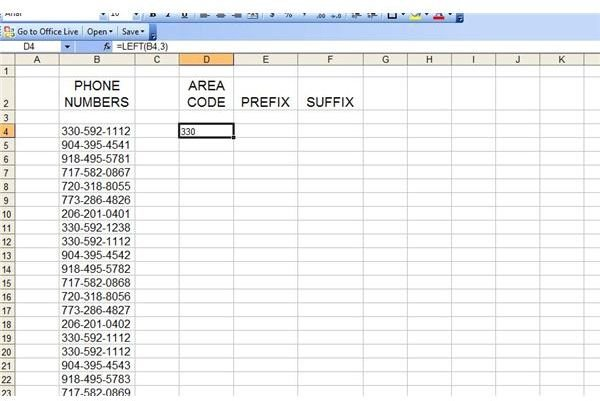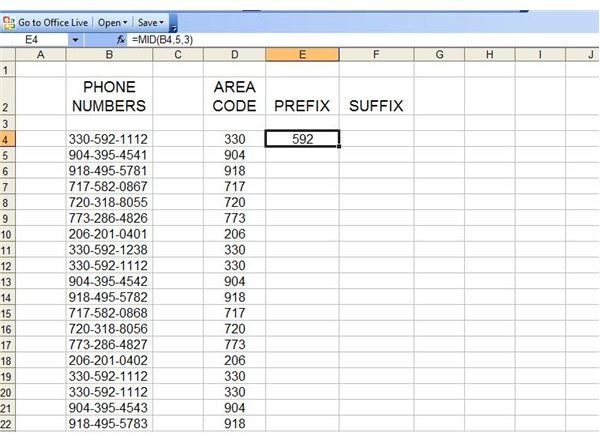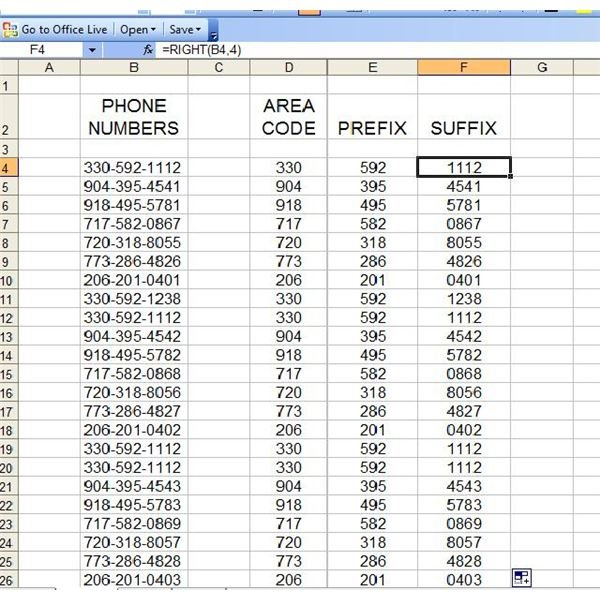# How to use Left, Right, and Mid Functions in Excel

Page content

## Isolating Parts of an Excel Cell

Excel’s LEFT, RIGHT and MID functions make it simple for you to slice up and use cell contents in other cells of a spreadsheet. For instance, perhaps you have imported a text file into Excel using .csv file format. (If you are unfamiliar with this process, check out the BrightHub tutorial How to Import CSV Files.) You can then use that text to create a database.

## Reformatting Excel Data

If you have a large text file in an Excel column that you need broken up and used in other cells, the LEFT, RIGHT and MID functions will make light work of it. This is especially true if all of the text is the same length.

This is because the syntaxes for LEFT, RIGHT and MID are as follows:

LEFT(text,num_chars)

RIGHT(text,num_chars)

MID(text,start_num,num_chars)

What this means is that Excel will count the number of characters you specify to “cut” and use the text in the cells to which the function is applied.

For example, we have a text list with numbers in ten-digit phone number format. We want to break down the list into an area code column, a prefix column, and a suffix column.

To begin, we will select the first cell in the Area Code column. In the Formula Bar, we will enter =LEFT( and Excel will prompt us for the text and number of characters. We know we want to draw the text from cell B4, and we also know we only want to use the first three characters from the left side of that cell. Therefore, the formula we enter will be =LEFT(B4,3).Next, we want to extract text from the first column and fill in the Prefix column. To begin, we will select the first cell in the Prefix column and go to the Formula Bar. Enter =MID( and Excel prompts us for the text, start number and number of characters we want to use. We know we want to extract the text from cell B4. We know the start number is “5” because we want to start with the fifth character (we don’t want to use the dash). Finally, we know that we want to extract three characters. Therefore, the formula for this cell will be =MID(B4,5,3).Finally, we want to extract the suffix from the text column. Just as with the previous instances, we’ll select the first cell and enter =RIGHT( into the Formula Bar. Excel will prompt us for the text source and the number of characters we want. For this cell, the formula will be =RIGHT(B4,4).

Once we have the formulas entered into each of the initial cells, we can select those three, grab the fill handle, and copy the formulas all the way down.If you have a different situation and need to combine text from different columns, check out this Bright Hub tutorial, Use Excel’s CONCATENATE Function to Combine Cells. Also, if you’re looking for more tips and tricks, be sure to take a look at the other Microsoft Excel function tutorials available.